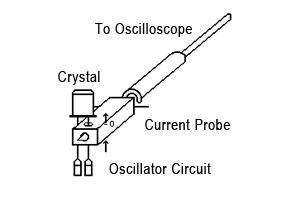# 在线技术服务

Q: What's the difference between OT and OT ultra low jitter?
Q: What is load capacitance (CL)?

CL stands for Load Capacitance. Load capacitance is defined as being the total capacitance present in the oscillator circuit as measured or calculated from the two terminals of the crystal. The output frequency of the crystal when assembled in an oscillator circuit is a function of CL. In order for the quartz crystal unit to exhibit an output frequency as close to specified as possible, it is important to measure precisely what the actual CL is in the circuit.

Q: The load capacitance (CL) of my resonant crystal is rated at 16pF. How do I calculate the value of the load capacitors used in my parallel resonant oscillator circuit?

The output frequency of the oscillator is a function of CL. If the crystal is rated at CL of 16pF, the specified frequency is optimized for a CL of 16pF in the oscillator circuit.

The CL can be estimated as follows:
CL=((C1 x Co) / (C1 + Co)) + Cstray

where Cstray is the stray capacitance in the circuit, typically 2-5pF, and Ci and Co are the external capacitors in the oscillator circuit. The CL in the circuit has to be as close to 16pF as possible to reach the specified output frequency. If the oscillation frequency is higher than specified, the capacitor values should be increased to lower the frequency. On the other hand, if the frequency is lower than specified, the capacitor values need to be decreased to raise the output frequency. When CL =16pF, C1 and Co will be approximately 22-30pF each, plus the additional stray capacitance. The values of C1 and Co used in the circuit will have great effect on the CL which influences the output frequency.

TAITIEN provides oscillator “Matching Test” to our customers to determine the right values of capacitors to use in the oscillator circuit to attain the nominal output frequency.

Q: What is PPM/PPB? How to determine PPM/PPB?

PPM stands for parts per million. PPB stands for parts per billion. In the context of oscillators, PPM/PPB is the ratio of frequency deviation with respect to nominal frequency. They are commonly used units in FCP industry to delineate the range of frequency variation in a crystal oscillator.

Example:
Nominal Frequency = 13.000000 MHz
Actual Frequency = 12.999974 MHz
=> (A.F. – N.F.) / N.F. = (12.999974-13.000000) / 13.000000
= – 0.000002
= – 2 x 10-6 (parts per million)
= – 2 PPM

Nominal Frequency = 20.000000000 MHz
Actual Frequency = 20.00000002 MHz
=> (A.F. – N.F.) / N.F. = (20.00000002-20.000000000) / 20.000000000
= + 0.000000001
= + 1 x 10-9 (parts per billion)
= + 1 PPB

Q: What is Drive Level? How to determine Drive Level?

Drive level is the power required from the oscillator circuit to operate/drive a crystal. The drive level can be calculated as follows:

Drive Level= I2 * RL, where I is the current going into the crystal unit and RL is the effective resistance of the crystal. Also, RL can be estimated as follows:
RL= RR(crystal impedance)*(1+C0/CL)^2,
C0: shunt capacitance of the crystal unit